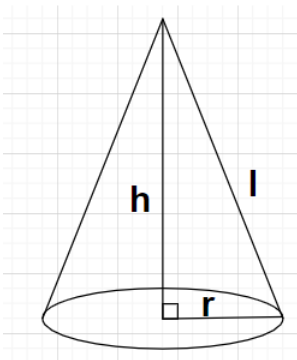Courses
Courses for Kids
Free study material
Free LIVE classes
MoreLIVE
Join Vedantu’s FREE Mastercalss

# The area of the curved surface of a cone is $60\pi {\text{ c}}{{\text{m}}^2}$. If the slant height of the cone is 8 cm, find the radius of the base.Verified
362.1k+ views
Hint: Here, we will be using the formula Curved surface area of the cone = $\pi rl$ where it is already given with the curved surface area and the slant height of the cone. This will lead to the value of the radius of the base of the cone.

Complete step by step solution:
Let r be the radius of the base of the cone.
Given, Curved surface area of the cone = $60\pi {\text{ c}}{{\text{m}}^2}$
Also given, Slant height of the cone l = 8 cm
As we know that the formula for the curved surface area of the cone with radius of the base as r and slant height as l is given by
Curved surface area of the cone = $\pi rl$
$\Rightarrow 60\pi = \pi r\left( 8 \right) \Rightarrow \dfrac{{60}}{8} = r \Rightarrow r = \dfrac{{15}}{2} = 7.5{\text{ cm}}$
Therefore, the radius of the base of the given cone is 7.5 cm.

Note: In these types of problems, if instead of the slant height of the cone we are given with the height of the cone. Then, we will evaluate the slant height of the cone (in terms of radius of base of the cone r) using the formula $l = \sqrt {{h^2} + {r^2}}$ and then use the formula for curved surface area of the cone.
Last updated date: 19th Sep 2023
Total views: 362.1k
Views today: 8.62k